# How to calculate number of days between two dates in Excel?

If the Excel user wants to know the number of days between two dates, Excel offers some powerful functions to find out the days between two dates. These functions usually help when you create a Gantt chart or timelines or calculate exact age. This tutorial is going to brief the methods to calculate the total days between two dates.

The number of days can be total days, weekend days, or working days between the two dates. In this chapter, we will use in-built or user-defined Excel formulas to calculate the days and brief you with several examples.

## Excel function to calculate days

Since we discussed that Excel offers some in-built functions to calculate the number of days, which are as follows-

• DAYS() – This function is used to calculate the difference between two dates as the number of days.
• DATEDIF() – This function is used to calculate the difference of two dates as the number of days, months, or years.

These functions, we are discussing in detail with their examples in this chapter below.

## What we are going to discuss –

In this chapter, we will cover the following topics to calculate the difference between two dates –

## DAYS() function

DAYS() is a date function of Excel that helps to calculate the difference between two dates as the number of days. This function usually needs two simple parameters, i.e., start date and end date.

“Number of days between 1 March, 2020 and 3 March 2020, the DAYS() function will return 2 days. But if you want to include both days to be counted, add 1 to the result.”

Syntax

This function requires only two arguments: start_date, end_date to get the days difference.

The start_date parameter holds a date from which the days to be start counted. At the same time, end_date keeps a date to end the count of days.

### Calculate days between two dates using DAYS()

We will take simple data in an Excel worksheet and apply this formula to get the difference between two dates using the DAYS() function.

Example 1

Step 1: We have a start date in A column and an end date in column B. See the following dataset below:Step 2: Select a cell (such as C2) to store the return value and write the following DAYS() formula in the formula bar or C2 cell itself.

=DAYS(B2,A2)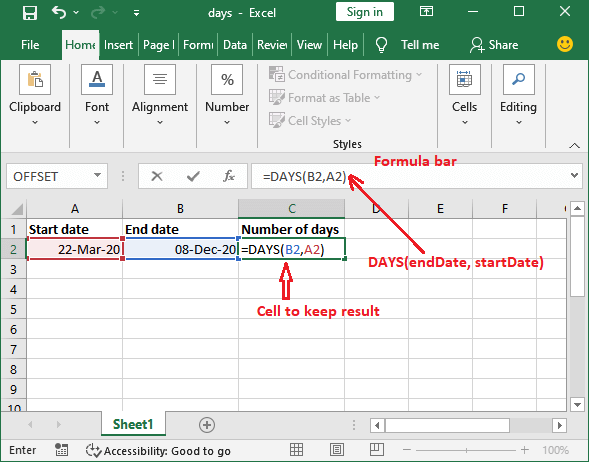Step 3: Hit the Enter key of the keyboard and get the calculated value as the number of days. There are 261 days between 22 March 2020 and 08 December 2020.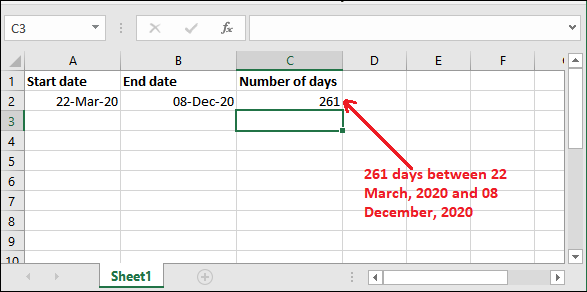Example 2

Step 4: The date format can be different, but the result will be consistent.Step 5: See for another data that the value does not conflict by changing the date format, and the result is consistent.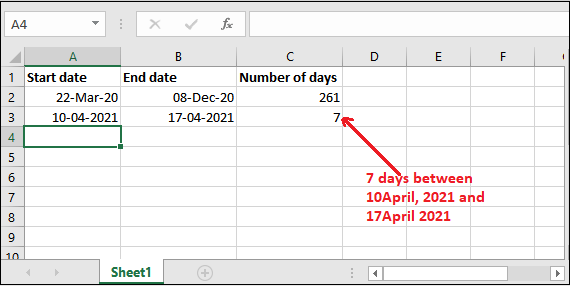It is a few steps simple process to get the number of days to be calculated. Now, we will use DATEDIF() formula.

Similarly, you can apply the same formula wherever you want to get the days between dates.

## DATEDIF() function

It is another date function of Excel. It allows the users to calculate the difference between two dates either in days, months, or years. It is an advanced method of DAYS() function and does more than it. It means that you can get the resultant value in the number of days, months, or years as well, whatever you want.

Syntax

This function accepts three arguments: start_date, end_date, and unit.

The first two parameters are self-explanatory and the third one is unit. It accepts the value to measure the difference between dates. It can be “D”, “M”, “Y” for days, months, and years respectively.

### Calculate days between two dates using DATEDIF()

Now, take an Excel worksheet and apply this formula to get the difference in the number of days between two dates using the DATEDIF() function.

Example 1

Step 1: We have a start date in A column and an end date in column B. See the following dataset below:Step 2: Select a cell (such as C2) to store the return value and write the following DATEDIF() formula in the formula bar or C2 cell itself.

=DATEDIF(A2,B2,”D”)

In this formula, we use “D” in the unit parameter to get the calculated result in days.Step 3: Hit the Enter key of the keyboard and get the calculated value as the number of days. There are 626 days between these two dates, 22 March 2020 and 08 December 2021.It is a few steps simple process like DAYS() to get the difference as days, months, or years. Now, we will use “M” and “Y” in unit parameter to get the result in months and years.

Step 4: Look for more outputs with the same start date and end date parameters but different unit values.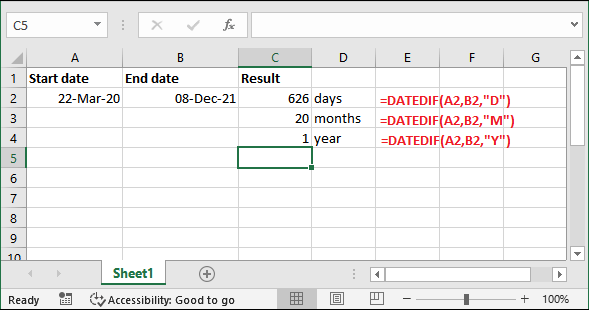### Combine the units in DATEDIF() function

Besides the single unit, you can also provide the combination of two units, e.g.., “MD”, “YD”, “YM”. The combination of two units provides a different type of result.

“MD” – It will return the number of days remaining in the past month only and ignore months and years data.

“YD” – It will return the number of days remaining in the past year and ignore months and years data.

“YM” – It will return the number of months remaining in the past year. It ignores days and years.

This type of combination of two units can be better understood with the help of a date difference example. Thus, we will provide these defined unit parameters in the below examples one by one.

### Remaining days in the past month

Write the following formula to get the days remaining in the past month and see the result.

=DATEDIF(A2,B2,”MD”)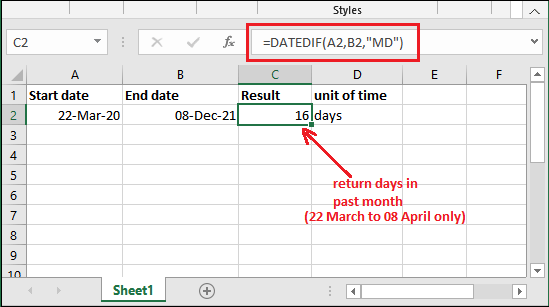Remember – the days return by using this unit parameter (“MD”), will never be more than 31 days. They will always be under 31 or 30 because a month can have maximum 31 days, not more than this.

### Return remaining days in past year

Write the following formula to get the days remaining in the past year and see the result.

=DATEDIF(A2,B2,”YD”)Remember – the days return by using this unit parameter (“YD”), will never be more than 364 days. They will always be under 365 because a year can have only 365 days. Leap year is an exceptional case.

### Return remaining months in past year

Write the following formula to get the total months remaining in past year and see the result.

=DATEDIF(A2,B2,”YM”)Remember – Whenever you will use this unit parameter (“YM”), it will never return more than 11 months. Since there can be only 12 months in a year. No matter how many years there are.

## Formula to find remaining days

We have a different formula to find the remaining days to complete another month or year. These remaining days used with other formula to get the dates difference in a format of year, months and days. This format makes easy to understand in case of exact age calculation.

It is a different type of formula that returns the remaining days instead of the total days. It requires only end date; see the syntax for it below –

Syntax

It will return the remaining days after a complete month or year calculation.

Let’s see this formula with an example and apply with the end date.

Step 1: We have a dates data where A2 cell is containing start date and B2 cell end date here.Step 2: We have created a new formula using endDate as given below.

Write a formula in the formula bar and execute it with your Excel data as showing below: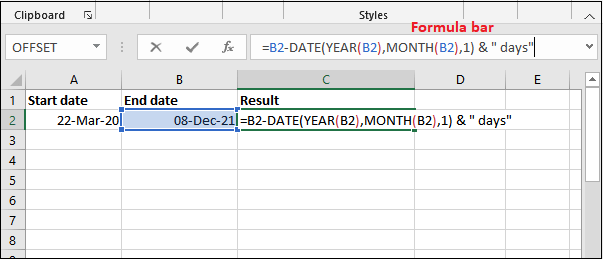Step 3: Hit the Enter key on your keyboard and get the calculated remaining days, i.e., 7 days.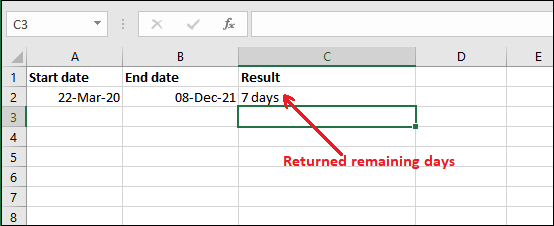This formula needs two more formulas to get the result in format (year, month, days). We will show you this in the next example. So, learn this carefully so that you can use it easily.

### Combine three formulas together and find the days in a format

By using the DAYS() and DATEDIF() formulas, you can only get the difference between two dates either in days, months, or years separately. But sometimes, you need to find the exact result in a format, e.g., 5 years, 2 months, 18 days. This format is usually required in age calculation and also many other places. So, we have a solution for it.

We can combine three formulas to achieve this result. For this, we need the following three formulas: DATEDIF() formula with a single unit, DATEDIF() with two units, and the remaining days formula.

For years: DATEDIF() formula with single unit

For remaining months: DATEDIF() formula with two units

For remaining days: Remaining days formula

Combine all three formulas using & operator to get a complete formula to find the formatted result in one go. Although it becomes a lengthy formula but provide exact result.

See an example for it as well.

Step 1: We have two dates of data where A2 cell is containing the start date and B2 cell end date here.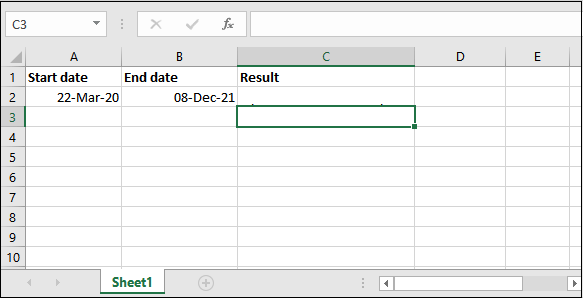Step 2: We have created a formula with three individual formulas by combining them using & (AND) operator.

You just provide the cell number accordingly and execute it with your Excel data as showing below: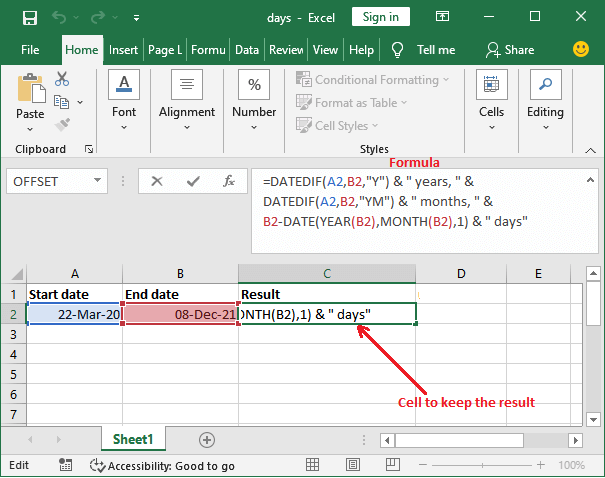Step 3: Hit the Enter key and get the result back in a format of year, month, and days.So, this format is more readable than 626 days, which is also equal to 1 year, 8 months, 7 days.